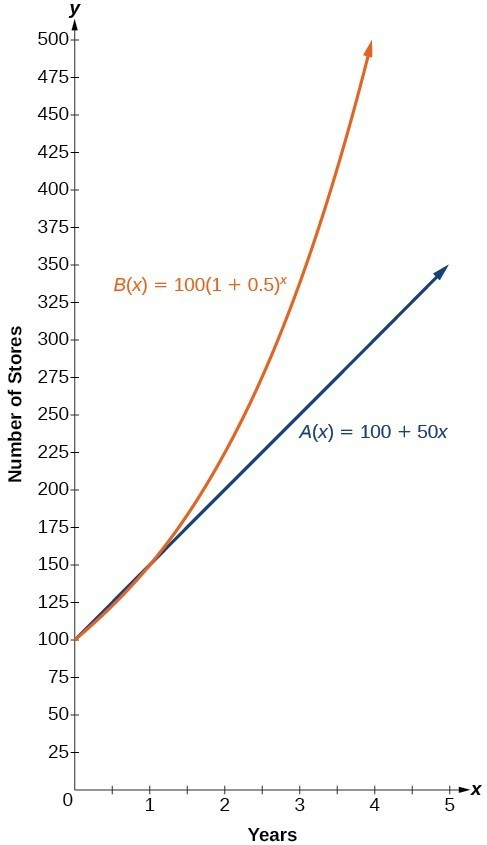## Evaluate exponential functions

Recall that the base of an exponential function must be a positive real number other than 1. Why do we limit the base b to positive values? To ensure that the outputs will be real numbers. Observe what happens if the base is not positive:

• Let = –9 and $x=\frac{1}{2}$. Then $f\left(x\right)=f\left(\frac{1}{2}\right)={\left(-9\right)}^{\frac{1}{2}}=\sqrt{-9}$, which is not a real number.

Why do we limit the base to positive values other than 1? Because base 1 results in the constant function. Observe what happens if the base is 1:

• Let = 1. Then $f\left(x\right)={1}^{x}=1$ for any value of x.

To evaluate an exponential function with the form $f\left(x\right)={b}^{x}$, we simply substitute x with the given value, and calculate the resulting power. For example:

Let $f\left(x\right)={2}^{x}$. What is $f\left(3\right)$?

$\begin{cases}f\left(x\right)\hfill & ={2}^{x}\hfill & \hfill \\ f\left(3\right)\hfill & ={2}^{3}\text{ }\hfill & \text{Substitute }x=3.\hfill \\ \hfill & =8\text{ }\hfill & \text{Evaluate the power}\text{.}\hfill \end{cases}$

To evaluate an exponential function with a form other than the basic form, it is important to follow the order of operations. For example:

Let $f\left(x\right)=30{\left(2\right)}^{x}$. What is $f\left(3\right)$?

$\begin{cases}f\left(x\right)\hfill & =30{\left(2\right)}^{x}\hfill & \hfill \\ f\left(3\right)\hfill & =30{\left(2\right)}^{3}\hfill & \text{Substitute }x=3.\hfill \\ \hfill & =30\left(8\right)\text{ }\hfill & \text{Simplify the power first}\text{.}\hfill \\ \hfill & =240\hfill & \text{Multiply}\text{.}\hfill \end{cases}$

Note that if the order of operations were not followed, the result would be incorrect:

$f\left(3\right)=30{\left(2\right)}^{3}\ne {60}^{3}=216,000$

### Example 1: Evaluating Exponential Functions

Let $f\left(x\right)=5{\left(3\right)}^{x+1}$. Evaluate $f\left(2\right)$ without using a calculator.

### Solution

Follow the order of operations. Be sure to pay attention to the parentheses.

$\begin{cases}f\left(x\right)\hfill & =5{\left(3\right)}^{x+1}\hfill & \hfill \\ f\left(2\right)\hfill & =5{\left(3\right)}^{2+1}\hfill & \text{Substitute }x=2.\hfill \\ \hfill & =5{\left(3\right)}^{3}\hfill & \text{Add the exponents}.\hfill \\ \hfill & =5\left(27\right)\hfill & \text{Simplify the power}\text{.}\hfill \\ \hfill & =135\hfill & \text{Multiply}\text{.}\hfill \end{cases}$

### Try It 1

Let $f\left(x\right)=8{\left(1.2\right)}^{x - 5}$. Evaluate $f\left(3\right)$ using a calculator. Round to four decimal places.

Solution

Because the output of exponential functions increases very rapidly, the term “exponential growth” is often used in everyday language to describe anything that grows or increases rapidly. However, exponential growth can be defined more precisely in a mathematical sense. If the growth rate is proportional to the amount present, the function models exponential growth.

### A General Note: Exponential Growth

A function that models exponential growth grows by a rate proportional to the amount present. For any real number x and any positive real numbers and b such that $b\ne 1$, an exponential growth function has the form

$\text{ }f\left(x\right)=a{b}^{x}$

where

• a is the initial or starting value of the function.
• b is the growth factor or growth multiplier per unit x.

In more general terms, we have an exponential function, in which a constant base is raised to a variable exponent. To differentiate between linear and exponential functions, let’s consider two companies, A and B. Company A has 100 stores and expands by opening 50 new stores a year, so its growth can be represented by the function $A\left(x\right)=100+50x$. Company B has 100 stores and expands by increasing the number of stores by 50% each year, so its growth can be represented by the function $B\left(x\right)=100{\left(1+0.5\right)}^{x}$.

A few years of growth for these companies are illustrated below.

Year, x Stores, Company A Stores, Company B
0 100 + 50(0) = 100 100(1 + 0.5)0 = 100
1 100 + 50(1) = 150 100(1 + 0.5)1 = 150
2 100 + 50(2) = 200 100(1 + 0.5)2 = 225
3 100 + 50(3) = 250 100(1 + 0.5)3 = 337.5
x A(x) = 100 + 50x B(x) = 100(1 + 0.5)x

The graphs comparing the number of stores for each company over a five-year period are shown in below. We can see that, with exponential growth, the number of stores increases much more rapidly than with linear growth.Figure 2. The graph shows the numbers of stores Companies A and B opened over a five-year period.

Notice that the domain for both functions is $\left[0,\infty \right)$, and the range for both functions is $\left[100,\infty \right)$. After year 1, Company B always has more stores than Company A.

Now we will turn our attention to the function representing the number of stores for Company B, $B\left(x\right)=100{\left(1+0.5\right)}^{x}$. In this exponential function, 100 represents the initial number of stores, 0.50 represents the growth rate, and $1+0.5=1.5$ represents the growth factor. Generalizing further, we can write this function as $B\left(x\right)=100{\left(1.5\right)}^{x}$, where 100 is the initial value, 1.5 is called the base, and x is called the exponent.

### Example 2: Evaluating a Real-World Exponential Model

At the beginning of this section, we learned that the population of India was about 1.25 billion in the year 2013, with an annual growth rate of about 1.2%. This situation is represented by the growth function $P\left(t\right)=1.25{\left(1.012\right)}^{t}$, where t is the number of years since 2013. To the nearest thousandth, what will the population of India be in 2031?

### Solution

To estimate the population in 2031, we evaluate the models for = 18, because 2031 is 18 years after 2013. Rounding to the nearest thousandth,

$P\left(18\right)=1.25{\left(1.012\right)}^{18}\approx 1.549$

There will be about 1.549 billion people in India in the year 2031.

### Try It 2

The population of China was about 1.39 billion in the year 2013, with an annual growth rate of about 0.6%. This situation is represented by the growth function $P\left(t\right)=1.39{\left(1.006\right)}^{t}$, where t is the number of years since 2013. To the nearest thousandth, what will the population of China be for the year 2031? How does this compare to the population prediction we made for India in Example 2?

Solution